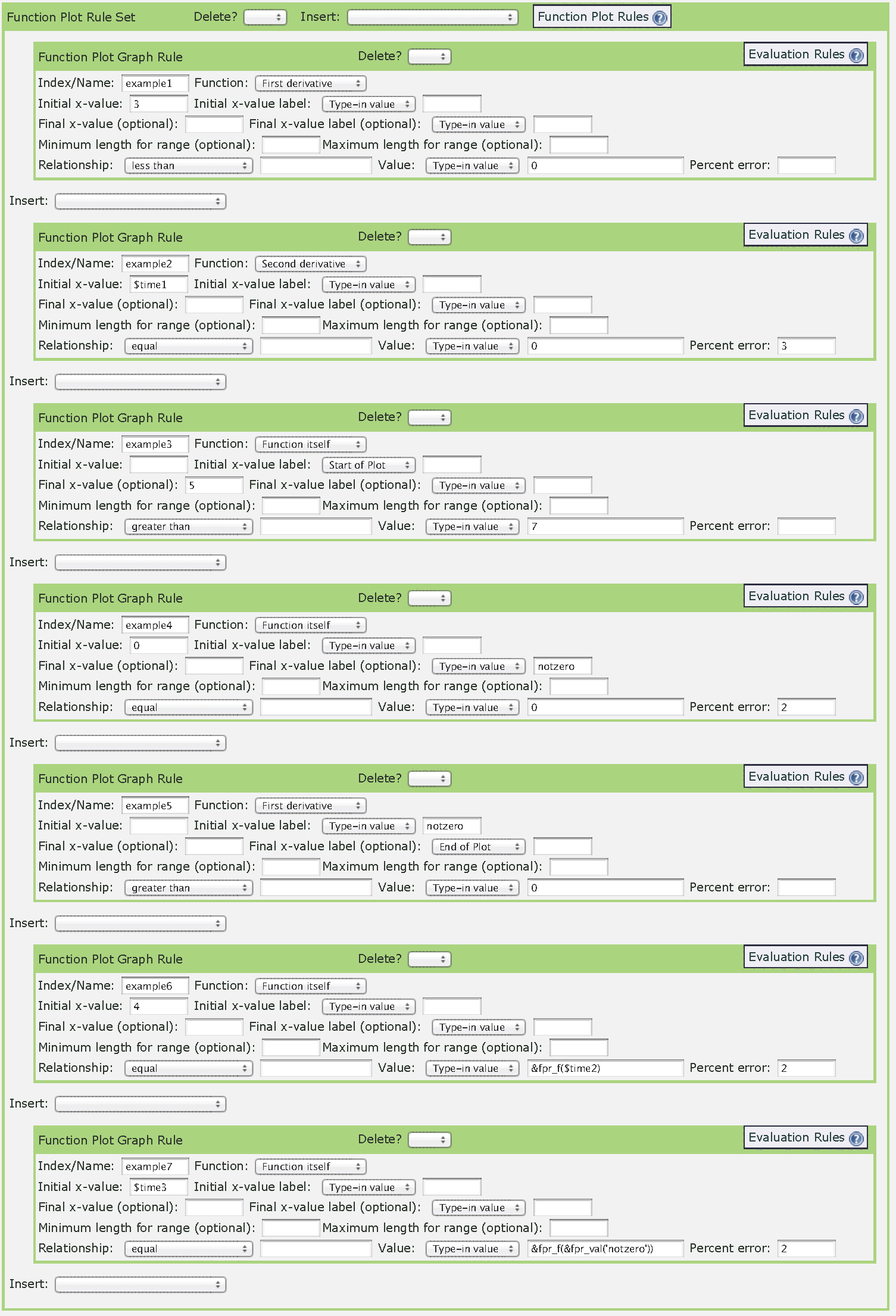###LON-CAPA Help

Overview - This box is used to create a rule that determines whether or not a submitted graph is correct. In general, it takes the form of testing the function, its integral, or its first or second derivative over a given set of x-values. The test can be to see if it equals, is greater than, or less than a specified value. Anywhere a number is needed, a variable can also be used. (Skip to the bottom for examples of rules.)

• "Index/Name" - This is an internal label for the rule. Something must be entered here, and it must be different for each rule. This same value will be used to add a conditional hint.

• "Function" - This determines what the server will be testing. For instance, choose 'First derivative' causes the server to evaluate the derivative of the entered answer over the given domain.

• "Initial x-value" and "Initial x-value label" - A value must be entered for one of these. Either choose a numerical value for x (the first option), or choose the beginning of the submitted answer, the end, or a previously chosen named point (see below).

• "Final x-value" and "Final x-value label" - This determines the end of the domain over which the rule examines. To test only a single point (the initial value), leave these blank. If a label is entered, such as 'positive', the point at which the rule fails will be given this special label. This label can then be used in subsequent rules as an 'Initial x-value label'.

• "Minimum length for range" - This tests that the difference between the initial and final x-values are at least a certain length apart. This is only useful if there is at least one label.

• "Maximum length for range" - This tests that the difference between the initial and final x-values are at most a certain length apart. This is only useful if there is at least one label.

• "Relationship" - The heart of the rule. This choice determines whether the chosen 'Function' is greater than, less than, equal to, etc. a certain 'Value'.

• "Value" - Enter the number you wish to compare to. It is also possible to choose 'not defined', in the event the answer should not have a value for the given domain. Within the value argument, the function itself can be evaluated using &fpr_f(), its derivative using &fpr_dfdx(), and its second derivative using &fpr_d2fdx2(). This allows for a comparison of two points on the graph. The value of a previously defined label can be retrieved using the function &fpr_val(), e.g., &fpr_val('positive'). Previous defined values from script blocks can also be retrieved as normal variables, e.g., \$x.

• "Percent error" - This allows for a margin of error in the y-direction. For instance, if the rule requires that the derivative be equal to 5, the server will accept values close enough to 5 that are within the percent error defined here. Note: Choosing 10% would not mean that the answer is correct as long as it is within the range 4.5-5.5. Instead, the percent corresponds to the total size of the graph. For the function itself, the 'percent error' is multiplied by the ymax-ymin; for the first derivative, it's multiplied by (ymax-ymin)/(xmax-xmin); for the second derivative, it's multiplied by (ymax-ymin)/(xmax-xmin)2; and for the integral, it's multiplied by (ymax-ymin)*(xmax-xmin).

The figure below shows some examples of rules.1. Checks that the derivative at x=3 is negative.

2. Checks that the second derivative at x=\$time1 is 0.

3. Checks that the value of the function > 7 from "Start of Graph" to x=5.

4. Checks that the function is 0 from x=0 until the function is no longer 0, labeling this new point 'notzero'.

5. Checks that the first derivative is positive between the point 'notzero' and the end of the graph.

6. Checks to see that the value of the function at x=4 is the same as the value of the function at \$time2. The Value here is &fpr_f(\$time2).

7. Checks that the value of the function at point 'notzero' is equal to the value of the function at x= \$time3. The Value here is &fpr_f(&fpr_val('notzero')).